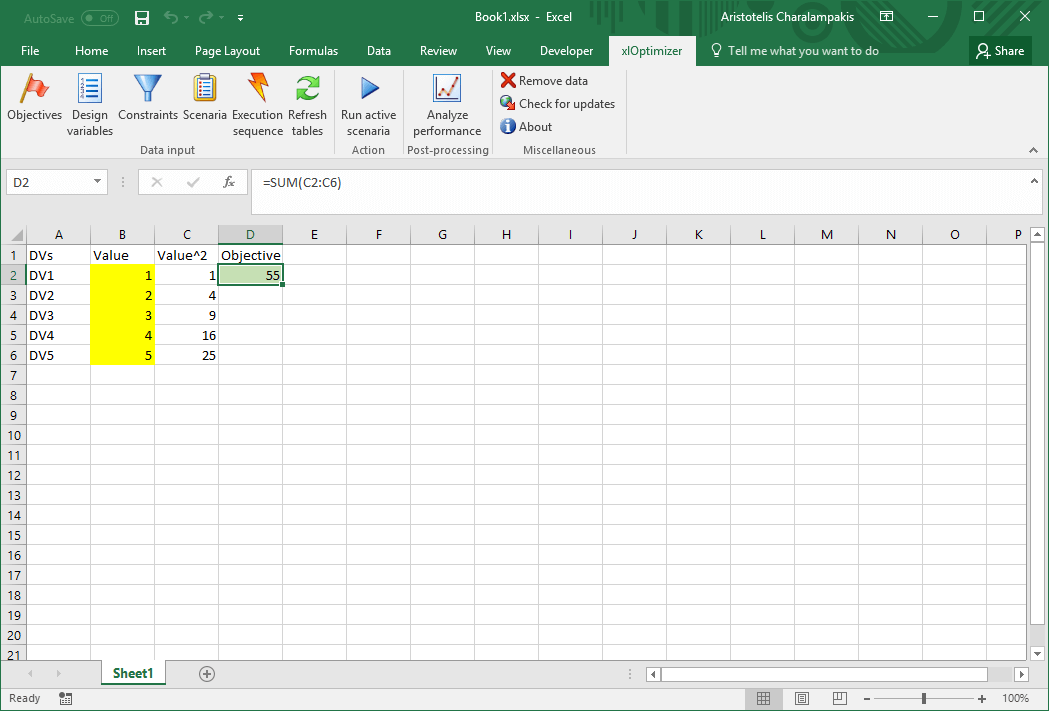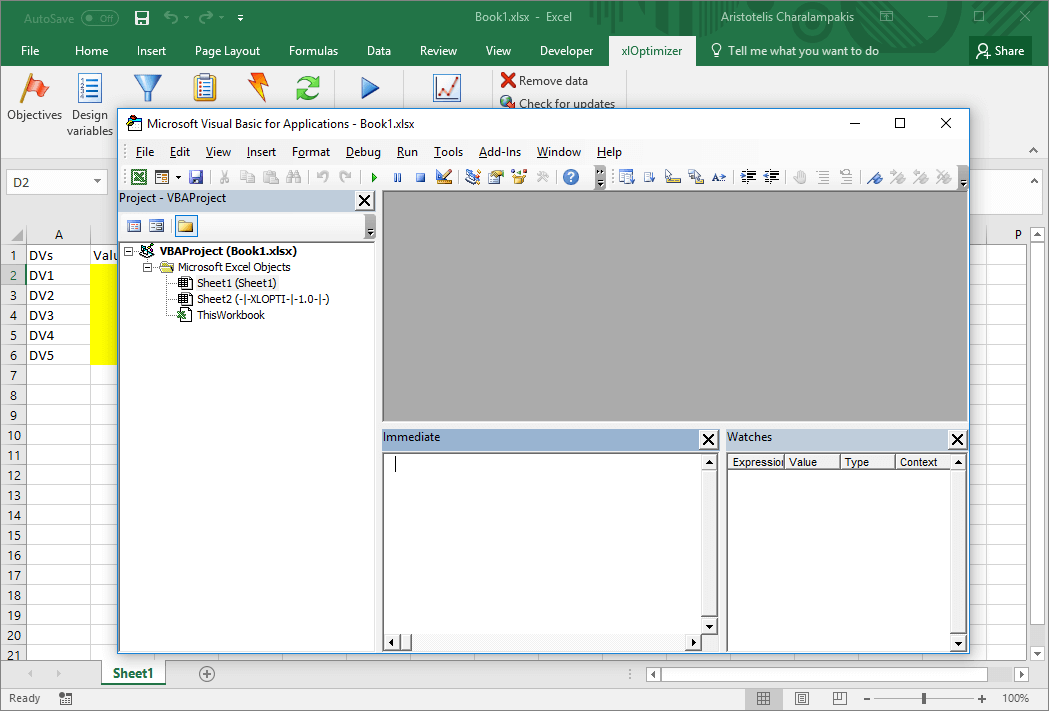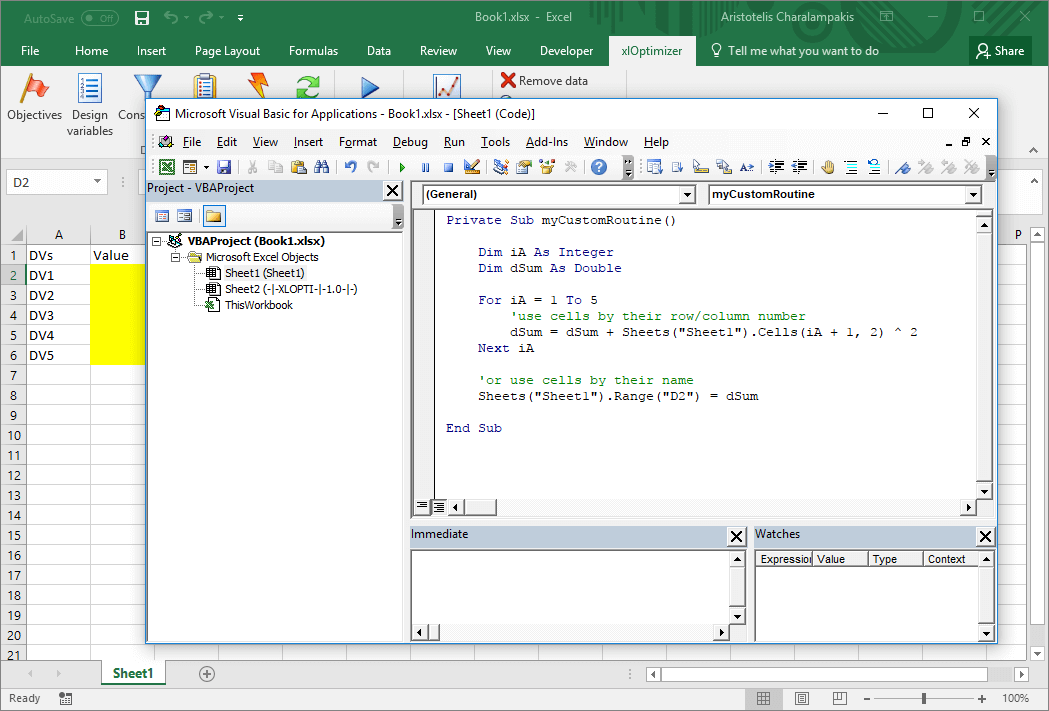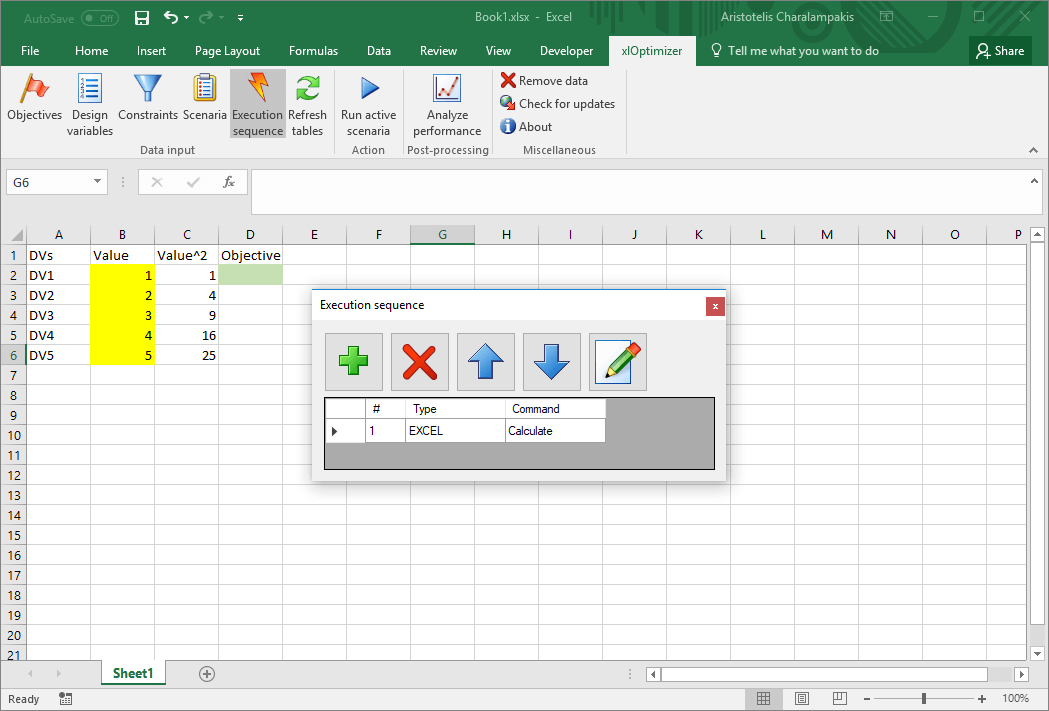## Introduction

The purpose of this example is the optimization (minimization) of the well-known sphere function using VBA (Visual Basic for Applications). VBA is a versatile tool which extends the capabilities of Microsoft Excel considerably. It is included in Microsoft Excel for free and provides the functionality of Visual Basic within the spreadsheet, which means that, among others, you can:

• insert standard controls such as command buttons, option buttons, etc and use them to execute your code
• insert custom subroutines to manipulate data
• use events raised by Microsoft Excel
• use external dlls (Dynamic Link Libraries), or even full executable programs, written by you or others, to perform difficult calculations, which are practically impossible to perform using Microsoft Excel alone!

In this very simple example, we will create a custom subroutine to execute our own code, i.e. evaluate the objective function, and set up xlOptimizer add-in so that it uses this subroutine instead of standard Microsoft Excel's calculation command.

This article assumes that you have read and understood the procedure regarding the minimization of sphere function using Microsoft Excel formulas only. The definition of the problem and the creation of the spreadsheet will not be repeated here.

## Step 1 : Setting-up the spreadsheet

We assume that you have already created the spreadsheet for the minimization of sphere function using Microsoft Excel formulas only:Now we will substitute the formula '=SUM(C2:C6)' with VBA code. Delete the formula by selecting cell D2 and pressing the Del button.

## Step 2 : Creating a custom routine

Press Alt+F11 to produce the VBA editor:On the treeview on the left, double-click on Sheet1. Then, on the top-right pane, write the following code to evaluate the sphere function:

 Dim iA As Integer
 Dim dSum As Double

 For iA = 1 To 5
   'use cells by their row/column number
   dSum = dSum + Sheets("Sheet1").Cells(iA + 1, 2) ^ 2
 Next iA

 'or use cells by their name
 Sheets("Sheet1").Range("D2") = dSum

The spreadsheet should look like this:Close the VBA editor window. We need to inform xlOptimizer that myCustomRoutine should be executed. In the xlOptimizer ribbon, click the Execution sequence button:By default, a single Calculate command is listed. This is a call to Microsoft Excel's internal calculation routine, which evaluates the formulas such as '=SUM(C2:C6)'. Since we do not need any such calculation, we can substitute this command with myCustomRoutine. Note that if our routine relied on some intermediate results obtained by Excel, we should not substitute the Calculate command, but rather add a new command. Therefore we need to build a sequence of actions (i.e. a list of actions, calls to internal calculations or custom routines) to be executed in each function evaluation by xlOptimizer.

In this case, we correct the single action to be a macro with the name 'Sheet1.myCustomRoutine':Press Ok to save the changes. That's it!

## Step 3 : Optimization

We can now proceed to the optimization by pressing the Run active scenaria button.

The file for this example can be downloaded here. Note that it is saved a macro enabled book with the extension .xlsm. You need to enable macros to run it.